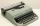# Find midpoint

FM=5y+13, MG=5-3y, FG=? M is the midpoint of FG. Use the given information to find the missing measure or value.

Result

x =  16

#### Solution:Leave us a comment of example and its solution (i.e. if it is still somewhat unclear...):

Showing 0 comments:Be the first to comment!#### To solve this example are needed these knowledge from mathematics:

Looking for help with calculating arithmetic mean? Looking for a statistical calculator? Do you have a linear equation or system of equations and looking for its solution? Or do you have quadratic equation?

## Next similar examples:

1. MG=7x-15,MG=7x-15, FG=33, x=? Point M is the midpoint of FG. Find unknown x.
2. Midpoint 6FM=8a+1, FG=42, a=? Point M is the midpoint of FG. Find unknown a.
3. CandyPeter had a sachet of candy. He wanted to share with his friends. If he gave them 30 candies, he would have 62 candies. If he gave them 40 candies, he would miss 8 candies. How many friends did Peter have?
4. EquationSolve the equation: 1/2-2/8 = 1/10; Write the result as a decimal number.
5. AverageThe arithmetic mean of the two numbers is 71.7. One number is 5. Calculate the second number.
6. Unknown numberIdentify unknown number which 1/5 is 40 greater than one tenth of that number.
7. Simple equationSolve for x: 3(x + 2) = x - 18
8. Arithmetic averageThe arithmetic mean of the five numbers is exactly 8. The sum of these four numbers is 30. What is the fifth number?
9. Boys and girlsThere are 48 children in the sports club, boys are 10 more than girls. How many girls go to the club?
10. Equation with xSolve the following equation: 2x- (8x + 1) - (x + 2) / 5 = 9
11. AssistantAssistant rewrote 15% of the entire manuscript in 12 hours. How many hours must still write to rewrite the rest of the manuscript?
12. Percentage - fractionsAbout what percentage we must increase number 1/6 to get number 1/3?
13. Equation with fractionsSolve equation: ? It is equation with fractions.
14. UN 1If we add to an unknown number his quarter, we get 210. Identify unknown number.
15. One-thirdA one-third of unknown number is equal to five times as great as the difference of the same unknown number and number 28. Determine the unknown number.
16. FactoryIn the factory workers work in three shifts. In the first inning operates half of all employees in the second inning and a third in the third inning 200 employees. How many employees work at the factory?
17. Cleaning windowsCleaning company has to wash all the windows of the school. The first day washes one-sixth of the windows of the school, the next day three more windows than the first day and the remaining 18 windows washes on the third day. Calculate how many windows ha